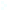Election CitationResearch Interests Name Bramson, Maury D. Location University of Minnesota Primary Field Applied Mathematical Sciences Secondary Field Mathematics Bramson has made seminal contributions to three distinct important areas of probability theory: (a) the KPP partial differential equation, (b) interacting particle systems, and (c) queueing networks. All three are motivated by, and have close connections to applied areas, such as traffic flow, telecommunications, tumor growth and population genetics. My research in probability theory mainly involves models from interacting particle systems, stochastic networks, and branching processes. Most of these models are motivated by phenomena or problems arising in mathematical physics, mathematical biology, engineering, and computer science. This includes the study of Gaussian free fields and the rate of decrease of the ~particle density for certain particle systems; the study of particle systems such as voter models and the contact process that are motivated by the movement of populations and spread of disease; and the study of the stability and large scale behavior of queueing networks, which includes the paradoxical behavior of queueing networks with slower input than service, which ~nonetheless may be unstable. ~